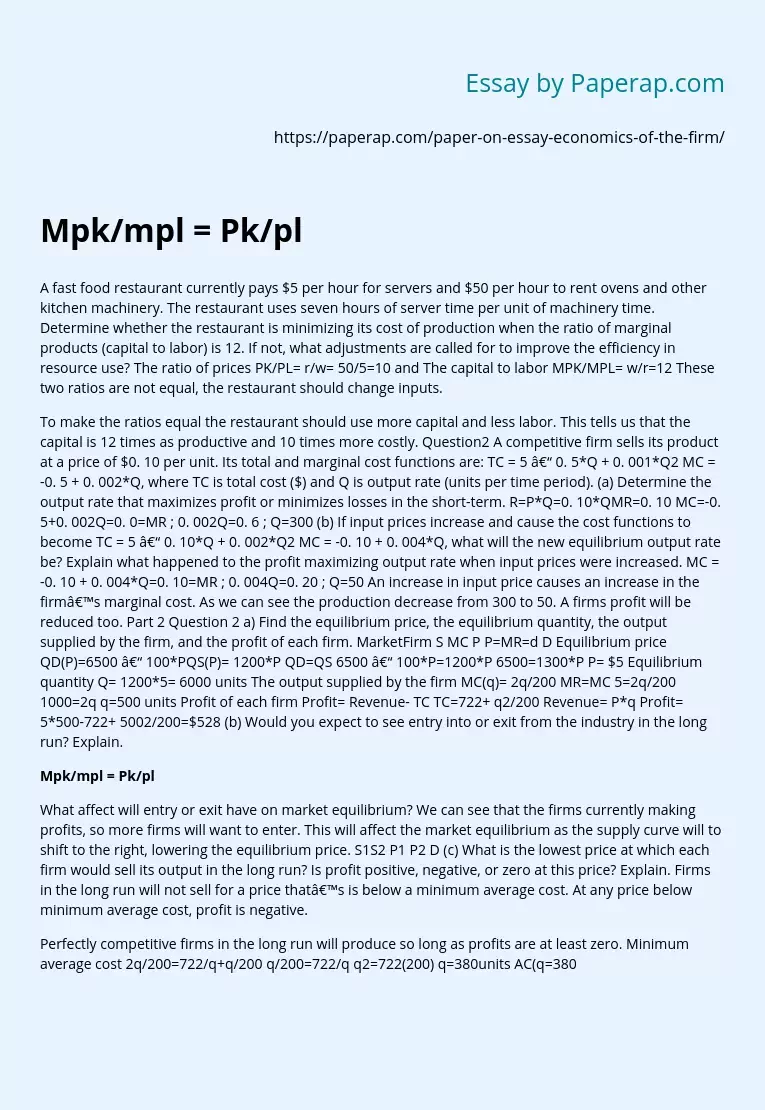# Calculations to Determine Firm Profit Mpk/mpl = Pk/pl

A fast food restaurant currently pays \$5 per hour for servers and \$50 per hour to rent ovens and other kitchen machinery. The restaurant uses seven hours of server time per unit of machinery time. Determine whether the restaurant is minimizing its cost of production when the ratio of marginal products (capital to labor) is 12. If not, what adjustments are called for to improve the efficiency in resource use? The ratio of prices PK/PL= r/w= 50/5=10 and The capital to labor MPK/MPL= w/r=12 These two ratios are not equal, the restaurant should change inputs.

To make the ratios equal the restaurant should use more capital and less labor. This tells us that the capital is 12 times as productive and 10 times more costly.

## Question2

A competitive firm sells its product at a price of \$0. 10 per unit. Its total and marginal cost functions are: TC = 5 – 0. 5*Q + 0. 001*Q2 MC = -0. 5 + 0. 002*Q, where TC is total cost (\$) and Q is output rate (units per time period).

(a) Determine the output rate that maximizes profit or minimizes losses in the short-term.

R=P*Q=0. 10*QMR=0. 10 MC=-0. 5+0. 002Q=0. 0=MR ; 0. 002Q=0. 6 ; Q=300 (b) If input prices increase and cause the cost functions to become TC = 5 – 0. 10*Q + 0. 002*Q2 MC = -0. 10 + 0. 004*Q, what will the new equilibrium output rate be? Explain what happened to the profit maximizing output rate when input prices were increased. MC = -0. 10 + 0. 004*Q=0. 10=MR ; 0. 004Q=0. 20 ; Q=50 An increase in input price causes an increase in the firm’s marginal cost. As we can see the production decrease from 300 to 50.

Get quality help nowBella HamiltonVerified

Proficient in: Economy5 (234)

“ Very organized ,I enjoyed and Loved every bit of our professional interaction ”+84 relevant experts are online

A firms profit will be reduced too.

### Part 2 Question 2

a) Find the equilibrium price, the equilibrium quantity, the output supplied by the firm, and the profit of each firm.

MarketFirm S MC P P=MR=d D Equilibrium price QD(P)=6500 – 100*PQS(P)= 1200*P QD=QS 6500 – 100*P=1200*P 6500=1300*P P= \$5 Equilibrium quantity Q= 1200*5= 6000 units The output supplied by the firm MC(q)= 2q/200 MR=MC 5=2q/200 1000=2q q=500 units Profit of each firm Profit= Revenue- TC TC=722+ q2/200 Revenue= P*q Profit= 5*500-722+ 5002/200=\$528

(b) Would you expect to see entry into or exit from the industry in the long run? Explain.

### What affect will entry or exit have on market equilibrium?

We can see that the firms currently making profits, so more firms will want to enter. This will affect the market equilibrium as the supply curve will to shift to the right, lowering the equilibrium price. S1S2 P1 P2 D

(c) What is the lowest price at which each firm would sell its output in the long run? Is profit positive, negative, or zero at this price? Explain.

Firms in the long run will not sell for a price that’s is below a minimum average cost. At any price below minimum average cost, profit is negative.

Perfectly competitive firms in the long run will produce so long as profits are at least zero. Minimum average cost 2q/200=722/q+q/200 q/200=722/q q2=722(200) q=380units AC(q=380)=\$3. 8 Therefore, the firm will not sell for any price less than \$3. 8 in the long run.

(d) What is the lowest price at which each firm would sell its output in the short run? Is profit positive, negative, or zero at this price? Explain.

The firm in a short run will sell for any positive price, as long as profit will not be negative and we know that negative profit is as price is below minimum average cost, or as long as price is bellow 3. 8.